# IOT Particle Temperature Sensor

Are you ever sitting in your room gaming with your friends and your room gets really warm? Our solution, an IOT temperature sensor!

IntermediateShowcase (no instructions)12 hours1,198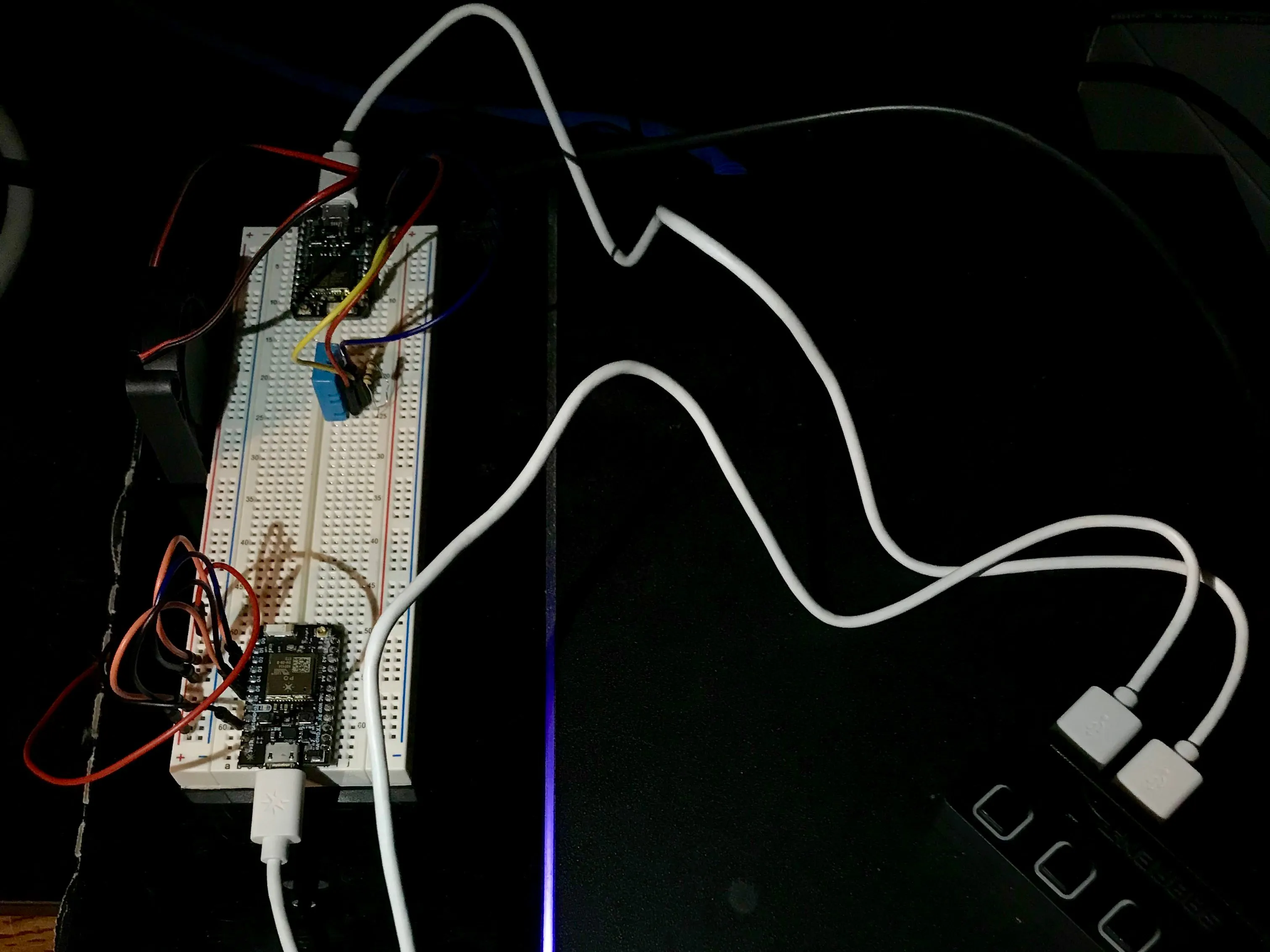## Things used in this project

### Hardware componentsParticle Photon
×2Resistor 10k ohm
×1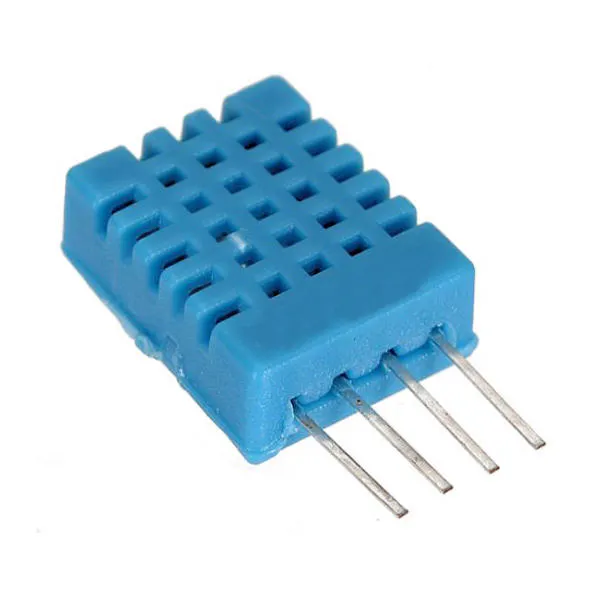DHT11 Temperature & Humidity Sensor (4 pins)
×1
 FAN
×1Jumper wires (generic)
×7

### Software apps and online services

 Particle IDE

## Schematics

### SetUp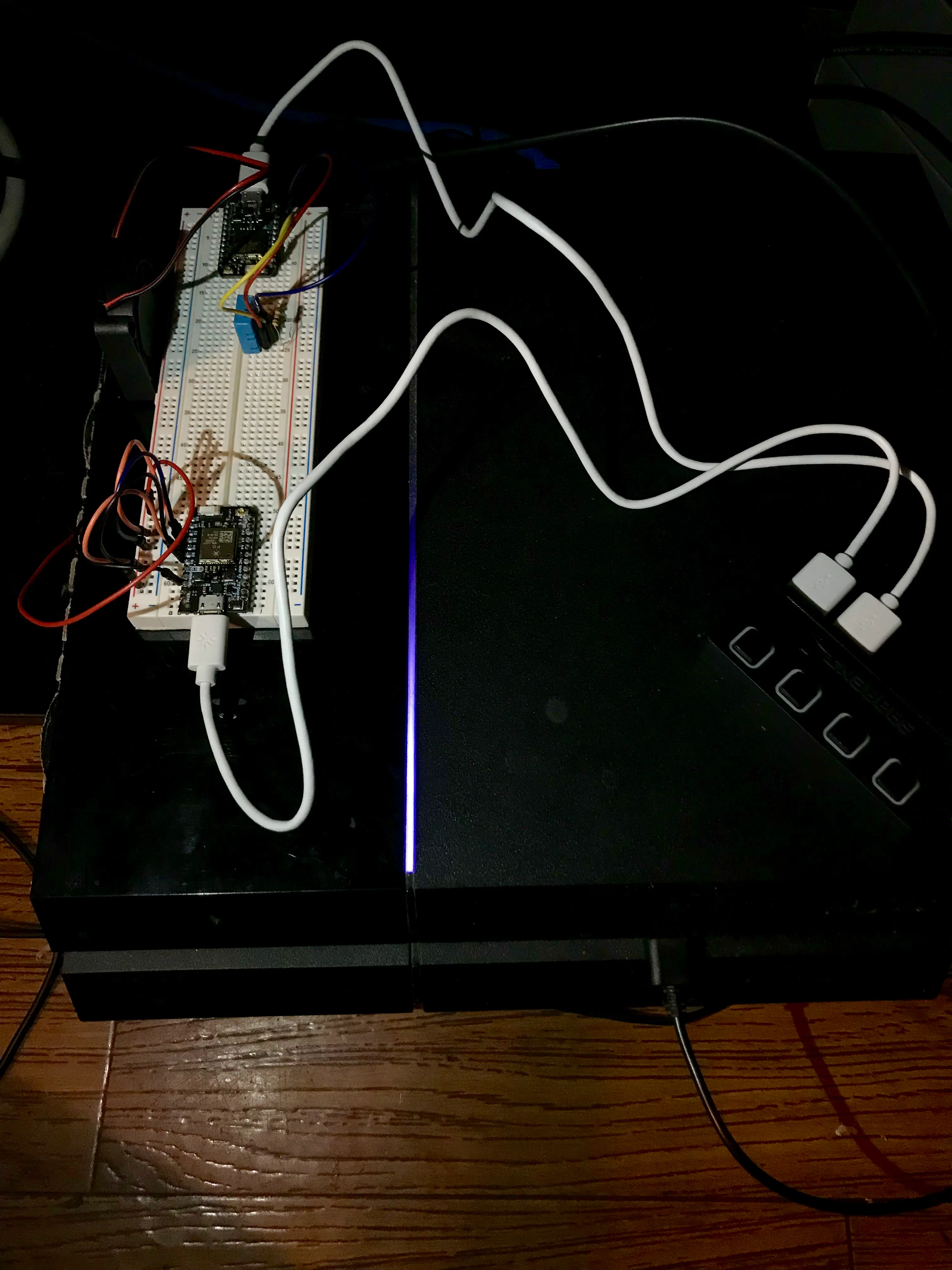### Circuit Diagram

These are the readings we received from the particle wired with the DHT11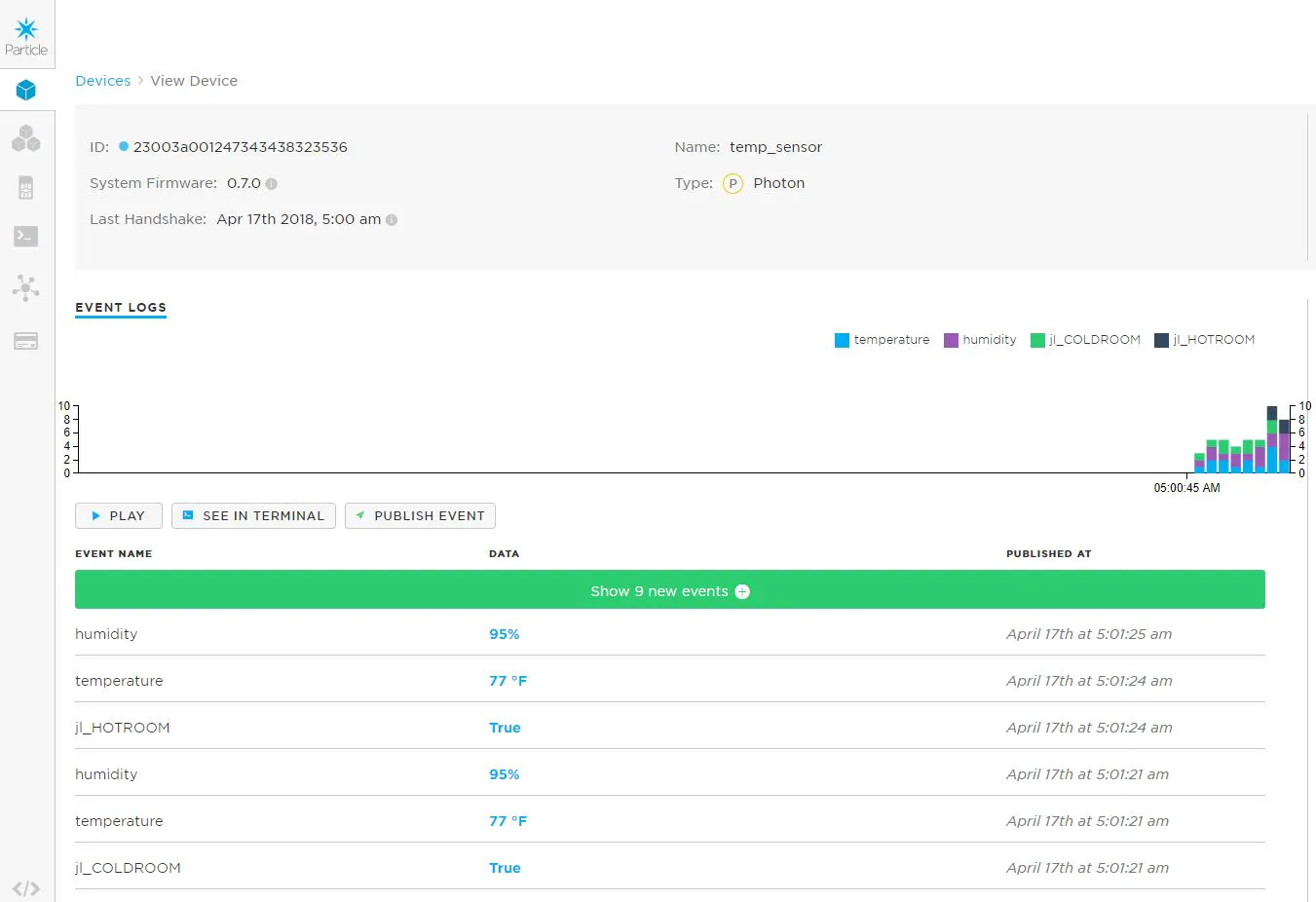### Circuit

This is a circuit diagram of how we wired both of the circuits.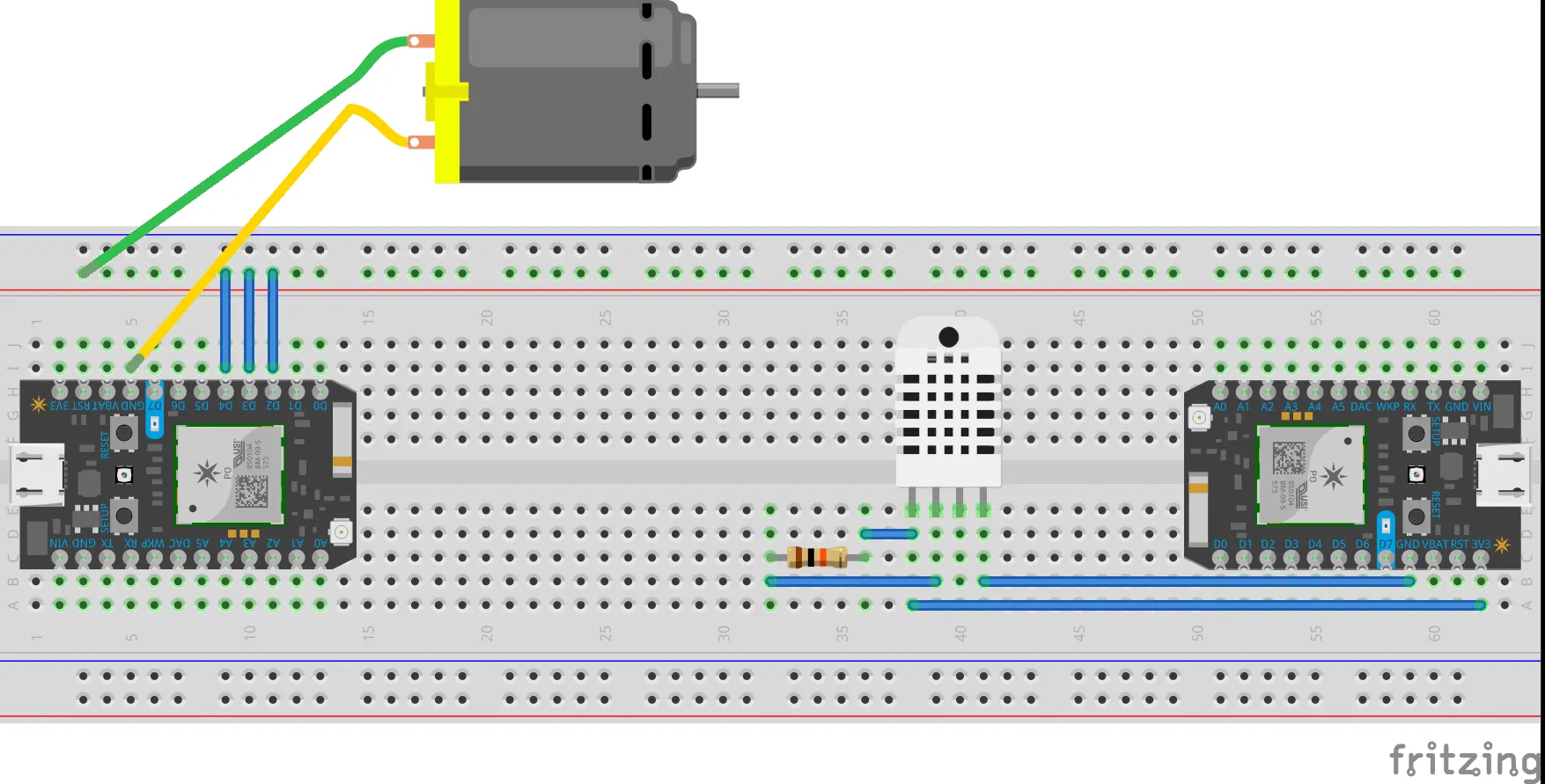## Code

### Temperature Sensor

C/C++
The coding below tells the photon to input the data from the DHT11 sensor. Depending on the temperature of the room the particle will publish either "jl_COLDROOM" or "jl_HOTROOM"
```// This #include statement was automatically added by the Particle IDE.

// DHT parameters
#define DHTPIN 2
#define DHTTYPE DHT11

// Variables
int temperature;
int humidity;
int LED=D7;

// DHT sensor
DHT dht(DHTPIN, DHTTYPE);

void setup() {
Particle.subscribe("jl_boardled", LEDON, "39002b000651353530373132");
Particle.subscribe("jl_boardledoff", LEDOFF, "39002b000651353530373132");
pinMode(LED,OUTPUT);
digitalWrite(LED,LOW);
// Start DHT sensor
dht.begin();
}

void LEDON(const char*event, const char *data){
digitalWrite(LED,HIGH);
}
void LEDOFF(const char*event, const char *data){
digitalWrite(LED,LOW);
}

void loop() {

// Humidity measurement
temperature = dht.getTempFarenheit();

// Humidity measurement
humidity = dht.getHumidity();

Particle.publish ("temperature", String(temperature) + " °F");
delay(500);
Particle.publish ("humidity", String(humidity) + "%");
delay(500);

String temp = String(temperature);
Particle.publish("temp", temp, PRIVATE);
delay(30000);

if( temperature > 80 ) {
// if condition is true then print the following
Particle.publish("jl_HOTROOM", "True");
} else {
// if condition is false then print the following
Particle.publish("jl_COLDROOM", "True");
}
}
```

### Fan

C/C++
This is the coding for the second particle. This particle is subscribed to the temperature sensors data and this determines weather power is delivered to the fan or not.
```int fan = D2;
int fan2 = D3;
int fan3 = D4;
int fan4 =D5;

void setup() {

Particle.subscribe("jl_HOTROOM", FANON, "23003a001247343438323536");
Particle.subscribe("jl_COLDROOM", FANOFF, "23003a001247343438323536");

pinMode(fan, OUTPUT);
digitalWrite(fan, LOW);
pinMode(fan2, OUTPUT);
digitalWrite(fan2, LOW);
pinMode(fan3, OUTPUT);
digitalWrite(fan3, LOW);
pinMode(fan4,OUTPUT);
digitalWrite(fan4,LOW);
}

void FANON(const char *event, const char *data){

digitalWrite(fan, HIGH);
digitalWrite(fan2, HIGH);
digitalWrite(fan3, HIGH);
digitalWrite(fan4,HIGH);
Particle.publish("jl_fanon", "1");
Particle.publish("jl_boardled","true");
}

void FANOFF(const char *event, const char *data){

digitalWrite(fan, LOW);
digitalWrite(fan2, LOW);
digitalWrite(fan3,LOW);
digitalWrite(fan4,LOW);
Particle.publish("jl_fanon", "0");
Particle.publish("jl_boardledoff","true");
}
```

C/C++
This code was uploaded from the particle library and makes the DHT11 function.
```/* DHT library
*
* modified for Spark Core by RussGrue
* */

DHT::DHT(uint8_t pin, uint8_t type, uint8_t count) {
_pin = pin;
_type = type;
_count = count;
}

void DHT::begin(void) {
// set up the pins!
pinMode(_pin, INPUT);
digitalWrite(_pin, HIGH);
}

float f;

switch (_type) {
case DHT11:
f = data;
return f;
case DHT22:
case DHT21:
f = data & 0x7F;
f *= 256;
f += data;
f /= 10;
if (data & 0x80)
f *= -1;
return f;
}
}
return NAN;
}

float DHT::getHumidity() {
}

float DHT::getTempCelcius() {
}

float DHT::getTempFarenheit() {
}

float DHT::getTempKelvin() {
}

float DHT::getHeatIndex() {
}

float DHT::getDewPoint() {
}

float DHT::convertFtoC(float f) {
return (f - 32) * 5 / 9;
}

float DHT::convertCtoF(float c) {
return c * 9 / 5 + 32;
}

float DHT::convertCtoK(float c) {
return c + 273.15;
}

float f;

switch (_type) {
case DHT11:
f = data;
return f;
case DHT22:
case DHT21:
f = data;
f *= 256;
f += data;
f /= 10;
return f;
}
}
return NAN;
}

float DHT::computeHeatIndex(float tempFahrenheit, float percentHumidity) {
// Wikipedia: http://en.wikipedia.org/wiki/Heat_index
return -42.379 +
2.04901523 * tempFahrenheit +
10.14333127 * percentHumidity +
-0.22475541 * tempFahrenheit * percentHumidity +
-0.00683783 * pow(tempFahrenheit, 2) +
-0.05481717 * pow(percentHumidity, 2) +
0.00122874 * pow(tempFahrenheit, 2) * percentHumidity +
0.00085282 * tempFahrenheit * pow(percentHumidity, 2) +
-0.00000199 * pow(tempFahrenheit, 2) * pow(percentHumidity, 2);
}

float DHT::computeDewPoint(float tempCelcius, float percentHumidity) {
double a = 17.271;
double b = 237.7;
double tC = (a * (float) tempCelcius) / (b + (float) tempCelcius) + log( (float) percentHumidity / 100);
double Td = (b * tC) / (a - tC);
return Td;
}

uint8_t laststate = HIGH;
uint8_t counter = 0;
uint8_t j = 0, i;
unsigned long currenttime;

// Check if sensor was read less than two seconds ago and return early
currenttime = millis();
// ie there was a rollover
}
return true; // return last correct measurement
//		delay(2000 - (currenttime - _lastreadtime));
}
/*
Serial.print("Currtime: "); Serial.print(currenttime);
*/

data = data = data = data = data = 0;

// pull the pin high and wait 250 milliseconds
digitalWrite(_pin, HIGH);
delay(250);

// now pull it low for ~20 milliseconds
pinMode(_pin, OUTPUT);
digitalWrite(_pin, LOW);
delay(20);
noInterrupts();
digitalWrite(_pin, HIGH);
delayMicroseconds(40);
pinMode(_pin, INPUT);

for ( i=0; i< MAXTIMINGS; i++) {
counter = 0;
counter++;
delayMicroseconds(1);
if (counter == 255) {
break;
}
}

if (counter == 255) break;

// ignore first 3 transitions
if ((i >= 4) && (i%2 == 0)) {
// shove each bit into the storage bytes
data[j/8] <<= 1;
if (counter > _count)
data[j/8] |= 1;
j++;
}

}

interrupts();

/*
Serial.println(j, DEC);
Serial.print(data, HEX); Serial.print(", ");
Serial.print(data, HEX); Serial.print(", ");
Serial.print(data, HEX); Serial.print(", ");
Serial.print(data, HEX); Serial.print(", ");
Serial.print(data, HEX); Serial.print(" =? ");
Serial.println(data + data + data + data, HEX);
*/

// check we read 40 bits and that the checksum matches
if ((j >= 40) &&
(data == ((data + data + data + data) & 0xFF)) ) {
return true;
}

return false;

}
```

C/C++
This code was uploaded from the particle library to get the DHT11 sensor to work.
```/* DHT library
*
* modified for Spark Core by RussGrue
* */

#ifndef DHT_H
#define DHT_H

#include "application.h"
#include "math.h"

// how many timing transitions we need to keep track of. 2 * number bits + extra
#define MAXTIMINGS 85

#define DHT11 11
#define DHT22 22
#define DHT21 21
#define AM2301 21

class DHT {
private:
uint8_t data;
uint8_t _pin, _type, _count;
float convertFtoC(float);
float convertCtoF(float);
float convertCtoK(float);
float computeHeatIndex(float tempFahrenheit, float percentHumidity);
float computeDewPoint(float tempCelcius, float percentHumidity);

public:
DHT(uint8_t pin, uint8_t type, uint8_t count=6);
void begin(void);
float getHumidity();
float getTempCelcius();
float getTempFarenheit();
float getTempKelvin();
float getHeatIndex();
float getDewPoint();

};
#endif
```

## Credits

### Jennifer Mericle

1 project • 0 followers

### Luke Honbarger

1 project • 0 followers